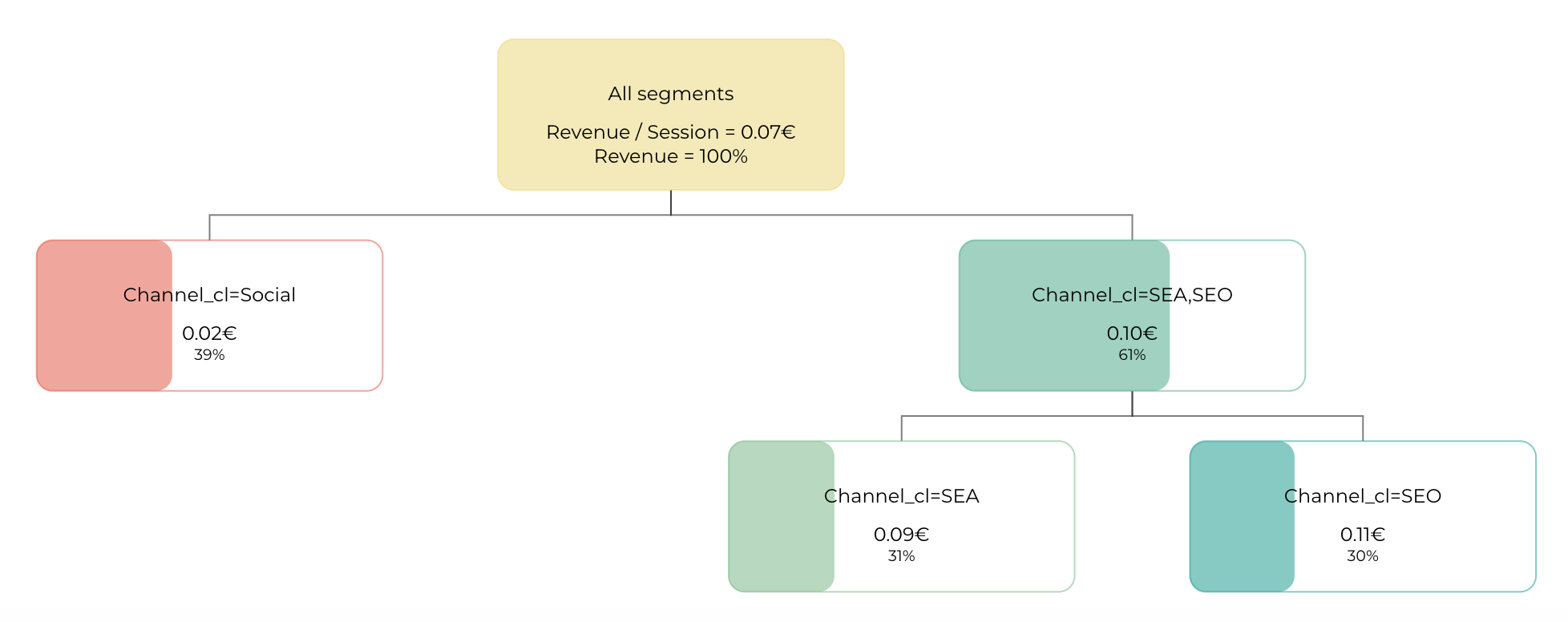# Pivot - Interface

Understand what is in the interface of DataMa PIVOT

Here is the main elements to find in DataMa PIVOT:

## Importance Chart

The dimension importance is the first element of the DataMa PIVOT interface. It sorts all the dimensions by importance from left to right, regarding the KPI you are following.

• Dimensions are sorted according to the Standard deviation which is weighted according to the volume. This basically means that dimensions on the left of the charts have segments that differ a lot on the selected KPIs. Depending on the dimension you’re looking at, one could then identify business opportunities in increasing the performance of low bubbles on this chart, if that is feasible.
• Size of the bubble represents the volume and height of the bubble represents the value of your KPI for that specific segment.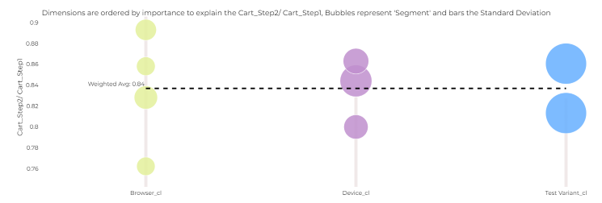Example: The AB Test example below shows the importance of 3 dimensions to measure the rate of transition to the next stage. Browser is the most important dimension and Test_variant is the least important. Each bubble of browser, represents a type of browser (Chrome, Firefox, Safari …), the gray bar represents the value of the Weighted Standard deviation used to sort the dimension.

## Simple chart

The bars show for each element of a dimension the contribution of this element to the global performance (primary numerator). You can change your dimension using the drop-down menu on the title of the slide. This is a non-aggregated view of the data. The black line is the average KPI of this element (ratio: numerator / denominator)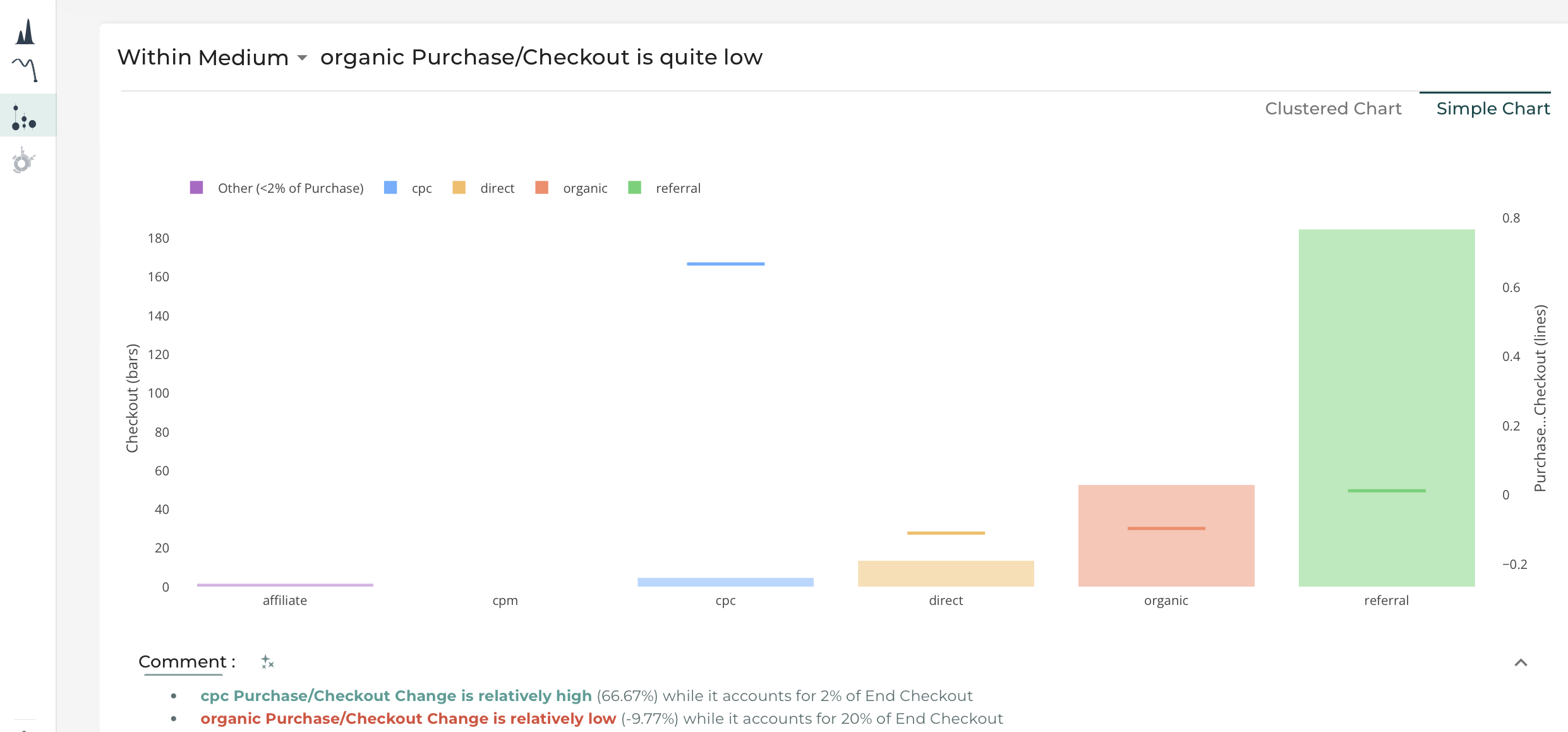## Clustered Chart

The bars show for each element of a dimension the contribution of this element to the global performance (primary numerator). You can change your dimension using the drop-down menu on the title of the slide. The black line is the average KPI of this element (ratio: numerator / denominator) Aggregation:

• For non-continuous elements, a cluster is created aggregating the element having a similar average KPI and representing less than “Aggregation in %” (see in the Settings menu)
• For continuous elements, multiple clusters are created aggregating elements having a similar average KPI.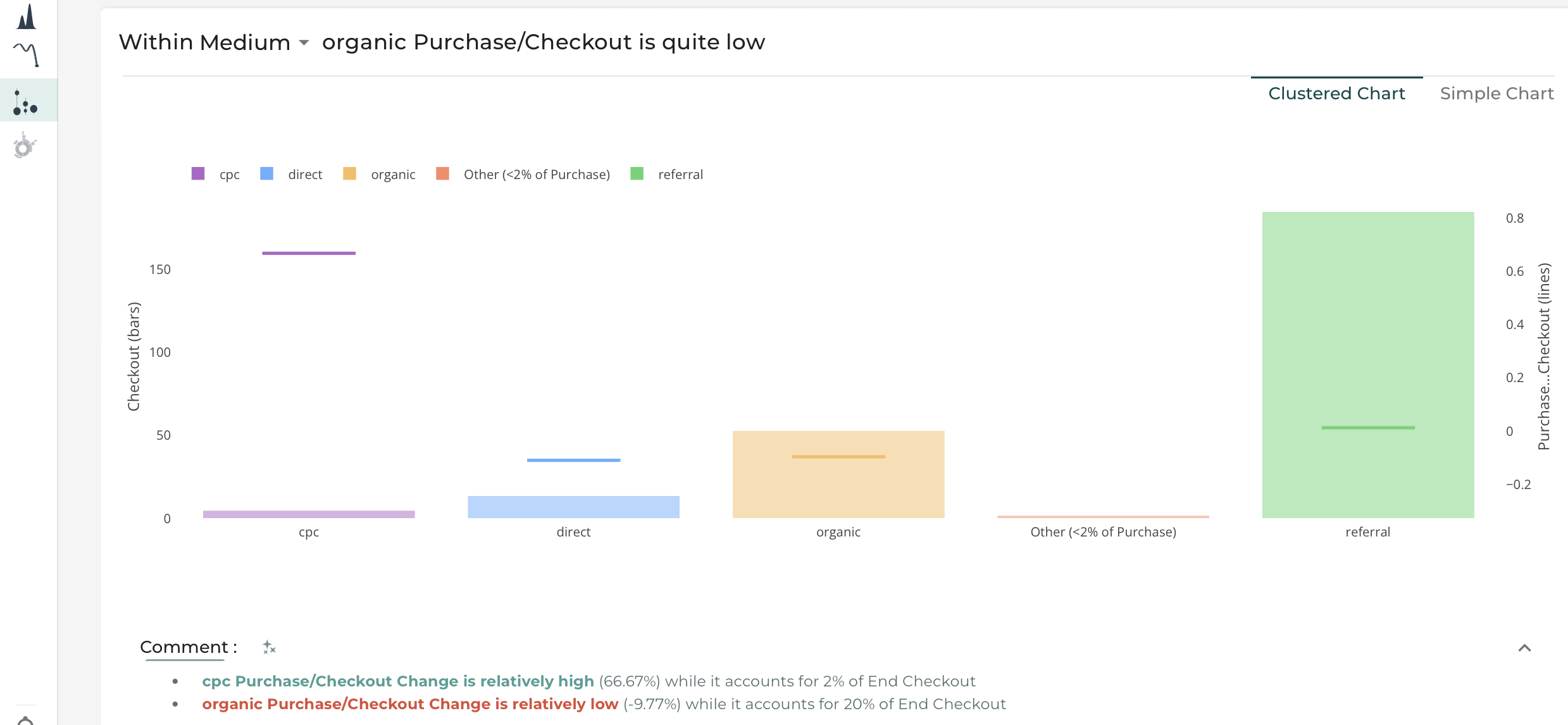## MariMekko

This aims to represent how one dimension is distributed in another dimension.

As for the Sankey diagram, you can change dimension by using the drop-down menu on the title. The first element corresponds to the splitted segment and the second one is the dimension you want to investigate (represented in each column).

## Sankey Diagram

This aims to discover how dimensions are correlated. Show the distribution between 2 dimensions, understand for 2 dimensions how the elements of these dimensions are distributed together. You can change dimension by using the drop-down menu on the title. The “% distrib” option allows you to represent the distribution by percentage instead of absolute value. This represents each element at the same size which helps you compare the distribution.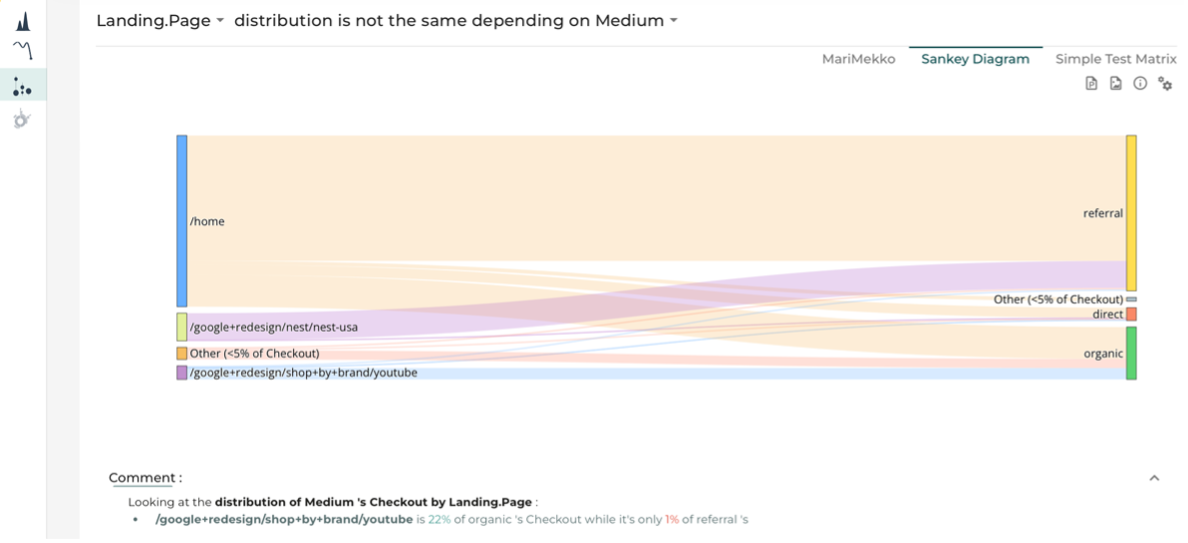The « % Distrib » option makes the segments on the right of the window all at the same size. This helps to compare segments between each other.

## Simple Test Matrix

The calculation is made using a simple correlation rate to determine whether dimensions are independent. Higher the score is, higher the correlation is. These figures are the percentage of correlation.When you click on one of the figures, it will filter automatically on the corresponding dimension (column and line).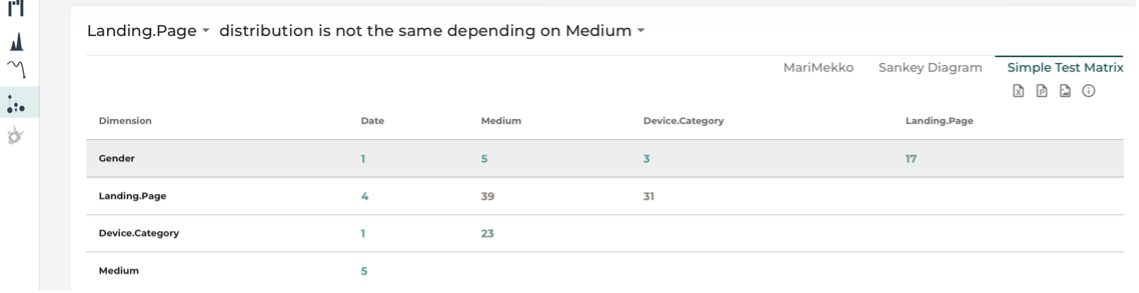## Decision TreeThe decision tree is based on a Rpart model (Recursive Partitioning and Regression Trees) Helps you identify the optimal characteristic of the sample that has the best performance. This decision tree helps you understand your dimensions and the importance they have explaining your performance. The figures in the square is the average KPI for this specific population and the percentage is the ratio of the population having this characteristic.

Steps of the decision tree are ordered according to the importance they have on the KPI. On the right find the more important dimension to follow and the worst on the left. In the box, see on the top the value of the KPI for the concerned population and on the bottom the percentage of population concerned by this selection.